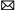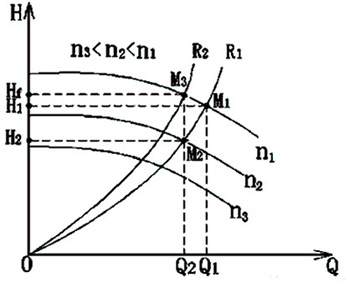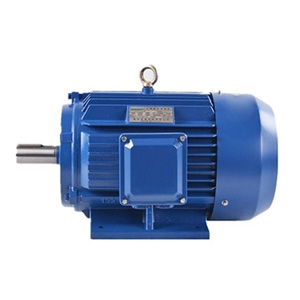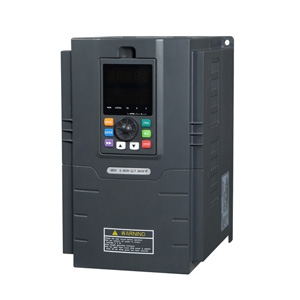Home » FAQ » How Does Variable Frequency Drive Control Motor Speed?

# How Does Variable Frequency Drive Control Motor Speed?

Why can the motor’s rotating speed change freely?
Unit of the motor’s rotating speed: r/min, number of revolution per minute, it can also be expressed as rpm.
For example: 2-pole motor, 50Hz 3000 r/min, 4-pole motor, 50Hz 1500r/min
Conclusion: The rotating speed of motor is proportional to the frequency.

The rotating speed of inductor type AC motor (hereinafter referred to as motor) is determined by the number of pole and frequency. Working principle of the motor determines that the number of pole is fixed and invariable. As the pole number is not a continuous number (times of 2, such as 2, 4, 6), it’s improper to regulate the motor speed by changing the pole number.
In addition, the frequency can be adjusted outside the motor and then supplied to the motor, so that the motor’s rotation speed can be freely controlled. Therefore, variable frequency drive with a purpose of frequency control is a preferred equipment for motor speed control.
n=60f/p
n: synchronous speed
f: power frequency
p: motor pole pairs
Conclusion: the optimal motor control method is to change frequency and voltage
If only the frequency is changed while the voltage is not changed, the motor may be burned out due to over-voltage (over-excitation) when the frequency is lowered. So the voltage shall also be changed while the motor frequency is changed. When the output frequency is above the rated frequency, the voltage can not continue to increase, the maximum voltage can only be equal to the rated voltage of the motor.

What will the output torque be when the motor’s rotating speed (frequency) changes?
Starting torque and maximum torque under the driving of VFD are smaller than those directly driven by power supply.
When the motor is powered by a power frequency supply, the starting and acceleration impact are huge. When the motor is powered by a VFD, the impact would be much weaker. Power frequency starting would produce a huge starting current. When a VFD is used, the output voltage and frequency of VFD are gradually added to the motor, so the starting current impact would be much smaller.
By using the flux vector controlled VFD, it will improve the deficiency of motor torque at low speed, or even in the low speed region, the motor can output enough torque.

When the VFD is Adjusted to a frequency higher than 50Hz or 60Hz, motor’s output torque will be reduced.
Common motors are designed and manufactured at a voltage of 50/60 Hertz, corresponding rated torques are also given within this voltage range. Therefore, the speed regulation at rated frequency is called constant torque speed regulation (T=Te, P<=Pe).
When the output frequency of VFD is greater than 50/60Hz, the torque produced by motor should reduce in a linear relationship inversely proportional to the frequency.
When the motor runs at a frequency higher than 50/60 Hz, the motor load must be taken into account to prevent the shortage of output torque of the motor.
For example, the torque produced by the motor at 100Hz is about 1/2 of that produced at 50Hz.
Therefore, the speed regulation above the rated frequency is called constant power speed regulation (P=Ue*Ie).

Applications of variable frequency drive above 50Hz
As everyone knows, the rated voltage and current of a specific motor is unchanged.
For example, the rated value of VFD and motor are 15kW/380V/30A, the motor can work above 50Hz.
When the rotation speed is 50Hz, output voltage and current of VFD are respectively 380V and 30A. If the output frequency is increased to 60Hz, the maximum output voltage and current of the VFD can only be 380V/30A. Obviously, the output power keeps the same, so we call it constant power speed regulation. What about the torque at this point?
As P=wT (w: angular velocity, T: torque).
As P keeps constant, w increases, the torque decreases correspondingly. We can analyze from another perspective:
Voltage of motor stator U=E+I*R (I refers to the current, R refers to the electronic resistance, E refers to the induced electromotive force)
It can be found that when the U and I keep constant, E also keeps constant. But E=k*f*X, (k: constant, f: frequency, X: flux), so X decreases correspondingly when f changes from 50 → 60Hz.
As for motor, T=K*I*X, (K: constant, I: current, X: flux), so the torque T decreases along with flux X decreases.
Meanwhile, when the frequency is below 50Hz, I*R is small, so when U/f=E/f keeps constant, the flux X is also a constant. Torque T is proportional to current. That’s why the over-current capability of VFD is usually used to describe its over-load (torque) capability. It’s thereof called constant torque speed regulation (rated current keeps unchanged → maximum torque keeps unchanged)
Conclusion: when the output frequency of VFD increases from above 50Hz, output torque of the motor decreases.

Plz Calculate (8 + 6) =
(Your comment will show after approved.)

You may also like:Variable Frequency Drivesinfo@variablefrequencydrive.net

Featured Articles
Variable Frequency Drive for FansFan is a kind of machine that compresses and conveys gas. If the pressure of exhausted air is quite low, the fan plays a role of ...
Variable Frequency Drive for Food ...Nowadays the food machinery industry has been rapidly developed. As the motor drive, variable frequency drive will change the ...
Can I Run a 60Hz Motor on 50Hz by ...We are often asked “can the motor with a rated frequency of 60Hz operate at 50Hz (vice versa)?” When the motor is operated at a ...
How Does Variable Frequency Drive ... The rotating speed of inductor type AC motor (hereinafter referred to as motor) is determined by the number of pole and ...
What is a Variable Frequency DriveVariable frequency drive (VFD) is an adjustable speed drive used for motor drive system, control the speed and torque of the AC ...